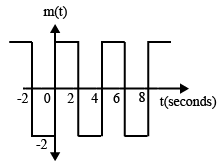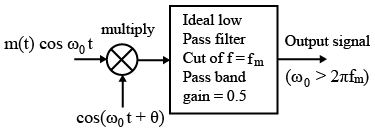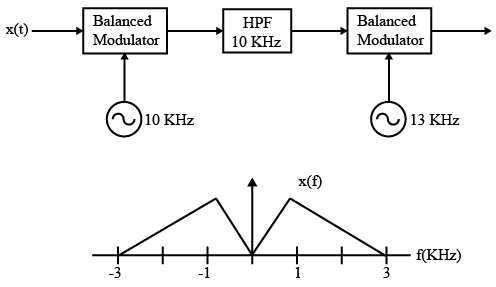# ISRO Scientist or Engineer Electronics May 2017

Instructions

For the following questions answer them individually

Question 1

# The signal m(t) as shown is applied both to a phase modulator (with kp as the phase constant) and a frequency modulator with (kf as the frequency constant) having the same carrier frequency. The ratio $$\frac{kp}{kf}$$ $$(in \frac{rad}{Hz})$$ for the same maximum phase deviation isQuestion 2

# A message m(t) bandlimited to the frequency fm has a power of Pm. The power of the output signal in the figure isQuestion 3

# The input x(t) and output y(t) of a system are related as $$y(t)=\int_{-\infty}^{t} x(t) \cos(4t) dt$$ The system isQuestion 4

# Consider a system shown in the figure. Let X(f ) and Y(f ) denote the Fourier transforms of x(t) and y(t) respectively. The ideal HPF has the cut-off frequency 10 KHz.The positive frequencies where Y(f ) has spectral peaks areQuestion 5

# Let $$x(t)=rect(t-\frac{1}{2})$$ where $$rect(t) = 1$$ for $$\frac{-1}{2} \leq t \leq \frac{1}{2}$$ and Zero otherwise , then the Fourier Transform of $$x(t) + x(-t)$$ will be given byQuestion 6

# A signal $$x(t) = sinc (\alpha t)$$, where $$\alpha$$ is a real constant, is the input to a Linear Time Invariant system whose impulse response is $$h(t) = sinc (\beta t)$$, where $$\beta$$ is a real constant. If min($$\alpha, \beta$$) denotes the minimum of $$\alpha$$ and $$\beta$$ and similarly, max($$\alpha, \beta$$) denotes the maximum of $$\alpha$$ and $$\beta$$ and K is a constant, which one of the following statements is true about the (here, $$sinc = \frac{\sin(\pi x)}{\pi x}$$) output of the system?Question 7

# A message signal given by $$m(t)=\frac{1}{2} \cos (\omega_{1} t)-(\frac{1}{2}) \sin (\omega_{2} t)$$  is amplitude modulated with a carrier of frequency $$\omega_{c}$$ to generates $$s(t) = [1 + m(t)] \cos( \omega_{c}t)$$.What is the power efficiency achieved by this modulation scheme?Question 8

# If $$E_{b}$$, the energy per bit of a binary digital signal, is $$10^{-5}$$ Ws(watt-Second) and the one-sided power spectral density of the white noise, $$N_{o}=10^{-6} \frac{W}{Hz}$$ then the output SNR of the matched filter isQuestion 9

# At a given probability of error, binary coherent FSK is inferior to binary coherent PSK by.Question 10

# If $$z_{0}$$ is a zero of a (real-valued) linear-phase FIR filter then following is/are also zero/zeros of a (real-valued) linear-phase FIR filter,OR